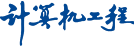##• 人工智能及识别技术 •

### 基于贝叶斯统计推断的离散分布估计算法

1. (太原科技大学复杂系统和计算智能实验室，太原 030024)
• 收稿日期:2012-04-17 出版日期:2013-08-15 发布日期:2013-08-13
• 作者简介:李 明(1985－)，男，硕士研究生，主研方向：智能计算；曾建潮，教授、博士生导师；何小娟，副教授、博士

### Discrete Distribution Estimation Algorithm Based on Bayesian Statistical Inference

LI Ming, ZENG Jian-chao, HE Xiao-juan

1. (Complex System and Computational Intelligence Laboratory, Taiyuan University of Science and Technology, Taiyuan 030024, China)
• Received:2012-04-17 Online:2013-08-15 Published:2013-08-13

Abstract: Bayesian statistical inference theory is added in the process of building the probability model of estimation of distribution algorithm. This paper proposes a discrete distribution estimation algorithm based on Bayesian statistical inference. A model of a priori probability is built according to the distributing regularity of the problem’s solution. The model of conditional probability is constructed through combining the probability model of advantage groups with forest structure of Bivariate Marginal Distribution Algorithm(BMDA). The model of posterior probability is given by combining the above mentioned probability model to guide new population generating. Simulation results show that the algorithm convergence rate is greater than the EDAs1 when solving the gr21 Traveling Salesman Problem(TSP). Analyzing the effect of combining speed and learning rate for this algorithm under the condition that parameters are fixed, such as population size and maximum running algebra etc. The results show when their values are 0.2, the algorithm performance is the most stable.